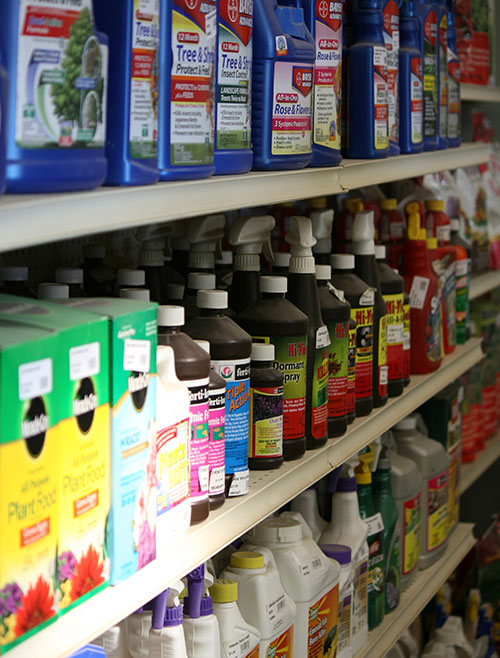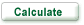# HARD GOODS

tools for the job -

##### HARDGOODS SEARCH:### NEW HARDGOODS ITEMS!

New Fertilizer and Weed Control!

View New Fertilizer and Weed Control (w/prices) - must be logged in.

Login or Register to become a Member

### Area Calculator

Area Calculator

#### How much area do you have?

 Square or Rectangle Length (ft.) Width (ft.)Circle Diameter (ft.)Triangle (that includes a right or 90° angle) -Measure length of two sides that meet at the right angle Side 1 (ft.)Side 2 (ft.)Area (Square feet):

### Mulch Calculator

Mulch Calculator

#### How much mulch do you need?

Depth:

Garden Size (sq. ft.):

You will need:

 2-cubic-foot bags or 3-cubic-foot bags or cubic feet or cubic yards or pickup truck loads (midsize)

THE CALCULATION:

Area (in square feet)  x  depth* of
mulch or compost you want to apply
(in inches) gives you the cubic feet needed.

*Depth factors to use:

1/8" = 0.0104166
1/4" = 0.020833
1/2" = 0.041666
1" = 0.08333
2" = 0.1666
3" = 0.25
4" = 0.333
5" = 0.415
6" = 0.498

Divide the cubic feet by 27 = cubic yards needed.

NOTE:

Total Area = (Length) X (Width)
- 27 cubic feet = 1 cubic yard
-There are 9 bags per yard.

### Grass Seed Calculator

Grass Seed Calculator

#### How Much Grass Seed?

Length (in ft)     Width (in ft)

THE CALCULATION:

Length x Width = Square Feet
Square Feet x .006 = lbs of seed required
(based on 200lbs of seed/acre, or 6 lbs per 1000 sq. ft.
-use half rate for overseeding)